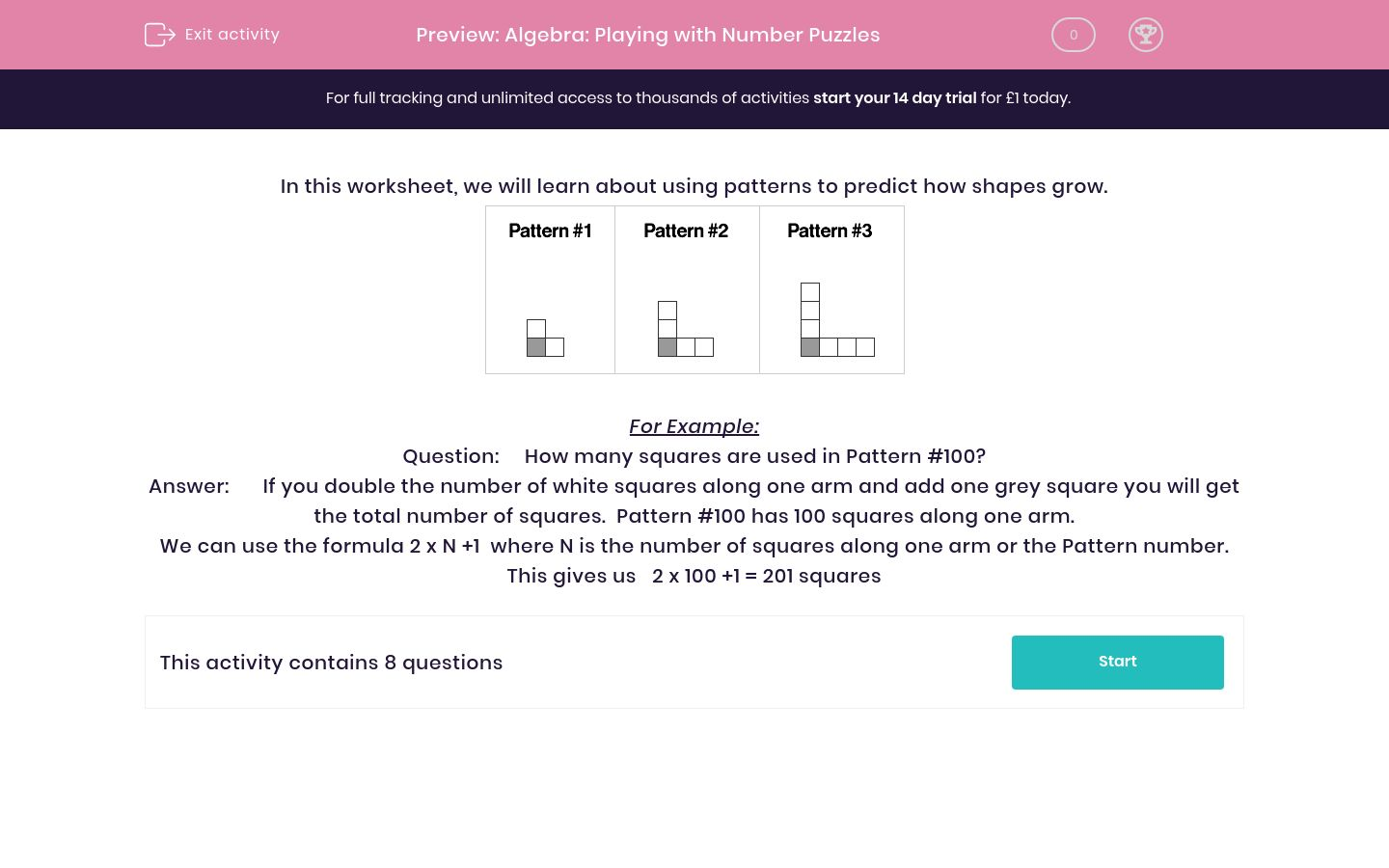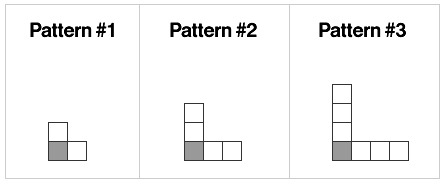# Algebra: Playing with Number Puzzles

In this worksheet, students solve number problems by working algebraically.Key stage:  KS 2

Curriculum topic:   Maths and Numerical Reasoning

Curriculum subtopic:   Equations and Algebra

Difficulty level:### QUESTION 1 of 10

In this worksheet, we will learn about using patterns to predict how shapes grow.For Example:

Question:     How many squares are used in Pattern #100?

Answer:       If you double the number of white squares along one arm and add one grey square you will get the total number of squares.  Pattern #100 has 100 squares along one arm.

We can use the formula 2 x N +1  where N is the number of squares along one arm or the Pattern number.

This gives us   2 x 100 +1 = 201 squaresHow many squares are in Pattern #21 ?How many squares are in Pattern #29 ?How many squares are in Pattern #72 ?How many squares are in Pattern #61 ?How many squares are in Pattern #53 ?How many squares are in Pattern #75 ?How many squares are in Pattern #86 ?How many squares are in Pattern #96 ?

• Question 1How many squares are in Pattern #21 ?

43
• Question 2How many squares are in Pattern #29 ?

59
• Question 3How many squares are in Pattern #72 ?

145
• Question 4How many squares are in Pattern #61 ?

123
• Question 5How many squares are in Pattern #53 ?

107
• Question 6How many squares are in Pattern #75 ?

151
• Question 7How many squares are in Pattern #86 ?

173
• Question 8How many squares are in Pattern #96 ?

193
---- OR ----

Sign up for a £1 trial so you can track and measure your child's progress on this activity.

### What is EdPlace?

We're your National Curriculum aligned online education content provider helping each child succeed in English, maths and science from year 1 to GCSE. With an EdPlace account you’ll be able to track and measure progress, helping each child achieve their best. We build confidence and attainment by personalising each child’s learning at a level that suits them.

Get started